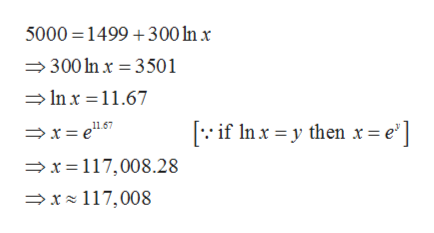# Using the model N(a), algebraically determine how much money needs to be spent on advertising in order to sell 5,000 units. N(a)=1499+300lnX

Question
21 views

Using the model N(a), algebraically determine how much money needs to be spent on advertising in order to sell 5,000 units.

N(a)=1499+300lnX

check_circle

Step 1

Given model is,

Step 2

Given that the number of units to be sell is 5000 units.

Therefore substi...help_outlineImage Transcriptionclose5000 1499 300 n x 300 In x 3501 Inx 11.67 if Inxy then r = e'] x=117,008.28 x 117,008 fullscreen

### Want to see the full answer?

See Solution

#### Want to see this answer and more?

Solutions are written by subject experts who are available 24/7. Questions are typically answered within 1 hour.*

See Solution
*Response times may vary by subject and question.
Tagged in

### Algebra